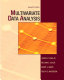### Multivariate Data Analysis

Data is displayed for academic year: 2023./2024.

#### Course Description

Multivariate data analysis forms one of the basic pillars of data science and is a generalization of univariate and bivariate statistical methods. Multivariate analysis is intended for simultaneous analysis and visualization of complex datasets with a large number of independent and/or dependent variables that are in different degrees of correlation, and their various effects cannot be interpreted separately. The contents of the course are grouped into three sections. The first part contains the basic concepts and basic techniques that precede the multivariate analysis, the second part relates to various advanced regression techniques and their understanding (with reference to high-dimensional data), and the third to techniques based on matrix decompositions (separation by eigenvalues ​​and separation by singular values).

#### Study Programmes

[FER3-EN] Data Science - profile
(2. semester)

#### Learning Outcomes

1. Define main notions in the multivariate data analysis
2. Explain mathematical backgrounds of main multivariate statistical procedures
3. Apply linear multiple regression analysis
4. Differentiate between principal component analysis and factor analysis
5. Justify the adequacy of different multivariate statistical methods for various problems
6. Interpret the results of multivariate statistical data analysis and explain their practical meaning

#### Forms of Teaching

Lectures

Lectures are given for 13 weeks in two two-hour sessions per week.

Exercises

Auditory exercises consist of solving practical examples and problems, and are integrated in the lecture sessions.

Laboratory

Programming assignments, demonstrated to the instructor or teaching assistant.

Continuous Assessment Exam
Laboratory Exercises 50 % 30 % 50 % 30 %
Mid Term Exam: Written 0 % 35 % 0 %
Final Exam: Written 0 % 35 %
Exam: Written 50 % 70 %
##### Comment:

The passing threshold is 50% of the total sum of points in the midterm and final exams.

#### Week by Week Schedule

1. Introductory concepts, statistical distance, sample geometry and random sampling
2. Random vectors and matrices, matrix decomposition, eigenvalues
3. Multivariate normal distribution
4. Statistical inference about vector means
5. Principal component analysis
6. Exploratory factor analysis
7. Multivariate linear regression and canonical correlation analysis
8. Midterm exam
9. Discriminant analysis
10. Clustering and distance methods
11. Correspondence analysis
12. Survival analysis
13. Time series analysis
14. The Lasso method for high dimensional data
15. Final exam

#### LiteratureRichard A. Johnson, Dean W. Wichern (2008.), Applied Multivariate Statistical Analysis, PearsonBarbara G. Tabachnick, Linda S. Fidell (2013.), Using Multivariate Statistics, PearsonJoseph F. Hair, William C. Black, Barry J. Babin, Rolph E. Anderson (2010.), Multivariate Data Analysis, Pearson

#### General

ID 222937
Summer semester
5 ECTS
L1 English Level
L1 e-Learning
45 Lectures
0 Seminar
15 Exercises
6 Laboratory exercises
0 Project laboratory